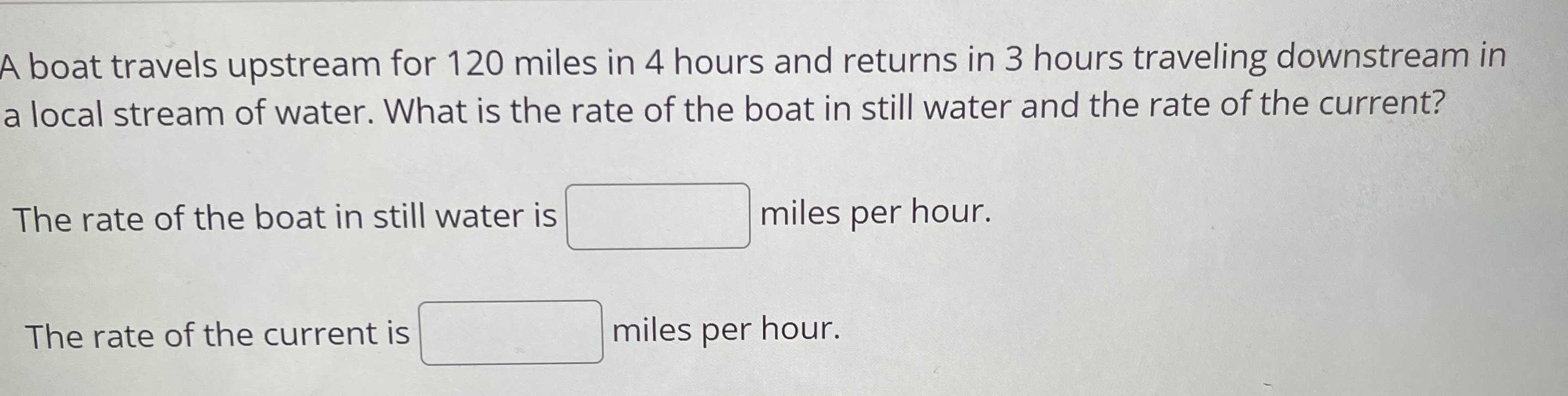### ¿Todavía tienes preguntas de matemáticas?

Pregunte a nuestros tutores expertos
Algebra
PreguntaA boat travels upstream for $$120$$ miles in $$4$$ hours and returns in $$3$$ hours traveling downstream in a local stream of water. What is the rate of the boat in still water and the rate of the current? The rate of the boat in still water is The rate of the current is

The rate of the boat in still water is: $$b = 35 \space mph$$
The rate of the current is: $$w = 5 \space mph$$